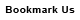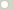# newton centimeter (Torque, Metric)

## 1 newton centimeter is equal to:Metric newton meter 0.01 dyne meter 1000 dyne centimeter 100000 kilogram-force meter 0.0010197162 kilogram-force centimeter 0.10197162 gram-force meter 1.0197162 gram-force centimeter 101.97162 British and U.S. long ton-force foot 3.2926882e-06 short ton-force foot 3.6878107e-06 pound-force foot 0.0073756215 pound-force inch 0.088507458 ounce-force inch 1.4161193ConversionsAreaCapacity and VolumeCircular measureComputer storageCurrencyDistance and LengthEnergy and WorkFuel ConsumptionMass and WeightPowerPressureSpeedTemperatureTimeTorqueSearchLink to us